# RD Sharma Solutions Class 9 Chapter 13 Linear Equations in Two Variables

Read RD Sharma Solutions Class 9 Chapter 13 Linear Equations in Two Variables below, students should study RD Sharma class 9 Mathematics available on Studiestoday.com with solved questions and answers. These chapter wise answers for class 9 Mathematics have been prepared by teacher of Grade 9. These RD Sharma class 9 Solutions have been designed as per the latest NCERT syllabus for class 9 and if practiced thoroughly can help you to score good marks in standard 9 Mathematics class tests and examinations

Exercise 13.1

Question 1: Express the following linear equations in the form ax + by + c = 0 and indicate the values of a, b and c in each case:
(i) -2x + 3y = 12
(ii) x – y/2 – 5 = 0
(iii) 2x + 3y = 9.35
(iv) 3x = -7y
(v) 2x + 3 = 0
(vi) y – 5 = 0
(vii) 4 = 3x
(viii) y = x/2
Solution:
(i) We have,
-2x + 3y = 12
We can write it as,
– 2x + 3y – 12 = 0
Given form is ax + by + c = 0,
Hence, the required values are
a = – 2
b = 3
c = -12

(ii) We have,
x – y/2 – 5= 0
Given form is ax + by + c = 0,
Hence, the required values are
a = 1
b = -1/2
c = -5

(iii) We have,
2x + 3y = 9.35
We can write it as,
2x + 3y – 9.35 = 0
Given form is ax + by + c = 0,
Hence, the required values are
a = 2
b = 3
c = -9.35

(iv) We have,
3x = -7y
We can write it as,
3x + 7y = 0
Given form is ax + by + c = 0,
Hence, the required values are
a = 3
b = 7
c = 0

(v) We have,
2x + 3 = 0
We can write it as,
2x + 0y + 3 = 0
Given form is ax + by + c = 0,
Hence, the required values are
a = 2
b = 0
c = 3

(vi) We have,
y – 5 = 0
We can write it as,
0x + y – 5 = 0
Given form is ax + by + c = 0,
Hence, the required values are
a = 0
b = 1
c = -5

(vii) We have,
4 = 3x
We can write it as,
3x + 0y – 4 = 0
Given form is ax + by + c = 0,
Hence, the required values are
a = 3
b = 0
c = -4

(viii) We have,
y = x/2
2y = x
x – 2y = 0
We can write it as,
x – 2y + 0 = 0
Given form is ax + by + c = 0,
Hence, the required values are
a = 1
b = -2
c = 0

Question 2: Write each of the following as an equation in two variables:
(i) 2x = -3
(ii) y = 3
(iii) 5x = 7/2
(iv) y = 3/2x
Solution:
(i) We have,
2x = -3
We can write the above equation in two variables as,
2x + 0y + 3 = 0

(ii) We have,
y = 3
We can write the above equation in two variables as,
0x + y – 3 = 0

(iii) We have,
5x = 7/2
We can write the above equation in two variables as,
5x + 0y – 7/2 = 0
Take LCM 2
(10x + 0y –7)/2 = 0
10x + 0y – 7 = 0

(iv) We have,
y = 3/2x
We can write the above equation in two variables as,
2y = 3x
3x – 2y = 0
We can write it as,
3x – 2y + 0 = 0

Question 3: The cost of ball pen is Rs 5 less than half of the cost of fountain pen. Write this statement as a linear equation in two variables.
Solution:
Let us assumed that the cost of a fountain pen be y
The cost of a ball pen be x
It is given that the cost of ball pen is Rs 5 less than half of the cost of fountain pen
x = y/2 − 5
by taking LCM 2
x = (y - 10)/2
2x = y – 10
2x – y + 10 = 0
Hence the required equation is 2x – y + 10 = 0.

Exercise 13.2

Question 1: Write two solutions for each of the following equations:
(i) 3x + 4y = 7
(ii) x = 6y
(iii) x + πy = 4
(iv) 2/3x – y = 4.
Solution:
(i) It is given that,
3x + 4y = 7
Put the value of x as 1.
3 × 1 + 4y = 7
3 + 4y = 7
4y = 7 - 3
4y = 4
y = 4/4
y = 1
Hence, the first solution is (1,1) x = 1 and y = 1.
Now, put the value of x as 2.
3 × 2 + 4y = 7
6 + 4y = 7
4y = 7 - 6
4y = 1
y = 1/4
Hence, the second solution is (2,  1/4) x = 2 and y = 1/4.

(ii) It is given that,
x = 6y
Put the value of x as 0.
0 = 6y
0/6 = y
y = 0
Hence, the first solution is (0, 0) x = 0 and y = 0.
Put the value of x as 6.
x = 6y
6 = 6y
6/6 = y
y = 1
Hence, the second solution is (6, 1) x = 6 and y = 1.

(iii) it is given that,
x + πy = 4
Put the value of x as 0.
0 + πy = 4
y = 4/π
Hence, the first solution is (0,4/π) x = 0 and y = 4/π.
Put the value of y as 0.
x + π × 0 = 4
x + 0 = 4
x = 4
Hence, the second solution is (0,4) x = 0 and y = 4.

(iv) It is given,
2/3x – y = 4
Put the value of x as 0.
2/3×0 – y = 4
– y = 4
y = –4
Hence, the first solution is (0,-4) x = 0 and y = -4.
Put the value of x as 3.
2/3 × 3 – y = 4
2 – y = 4
2 – y = 4 – 2
– y = 4 – 2
y = -2
Hence, the second solution is (3,-2) x = 3 and y = -2.

Question 2: Write two solutions of the form x = 0, y = a and x = b, y = 0 for each of the following equations:
(i) 5x – 2y =10
(ii) -4x + 3y =12
(iii) 2x + 3y = 24
Solution:
(i) It is given that,
5x – 2y = 10
Put the value of x = 0.
5 × 0 – 2y = 10
– 2y = 10
– y = 10/2
y = – 5
Hence, the solution of 5x - 2y = 10 is x = 0 and y = -5.

Put the value of y = 0
5x – 2 x 0 = 10
5x = 10
x = 2
Hence, the solution of 5x – 2y = 10 is x =2 and y = 0.

(ii) It is given that,
– 4x + 3y = 12
Put the value of x = 0
– 4 × 0 + 3y = 12
3y = 12
y = 4
Hence, the solution of -4x + 3y = 12 is x = 0 and y = 4.

Put the value of y = 0
– 4x + 3 × (0) = 12
– 4x = 12
x = -12/4
x = -3
Hence, the solution of -4x + 3y = 12 is x = -3 and y = 0.

(iii) Given, 2x + 3y = 24
Put the value of x = 0
2 × (0) + 3y = 24
3y = 24
y = 24/3
y = 8
Hence, the solution of 2x+ 3y = 24 is x = 0 and y = 8.

Put the value of y = 0
2x + 3 × (0) = 24
2x = 24
x = 24/2
x =12
Hence, the solution of 2x + 3y = 24 is x = 12 and y = 0.

Question 3: Check which of the following are solutions of the equation 2x – y = 6 and which are not:
(i) (3 , 0)
(ii) (0 , 6)
(iii) (2 , -2)
(iv) (√3, 0)
(v) (1/2,-5)
Solution:
(i) (3, 0)
Here x = 3 and y = 0
Put the values of x and y in given equation.
2x – y = 6
2(3) – (0) = 6
6 = 6
(3,0) is solution of 2x – y = 6. Hence, the statement is true.

(ii) (0, 6)
Here x = 0 and y = 6
Put the values of x and y in given equation.
2x – y = 6
2 × (0) – 6 = 6
-6 = 6
(0, 6) is not a solution of 2x – y = 6. Hence, the statement is false.

(iii) (2, -2)
Here x = 0 and y = 6
Put the values of x and y in given equation.
2x – y = 6
2 × (2) – (– 2) = 6
4 + 2 = 6
6 = 6
(2,-2) is a solution of 2x – y = 6. Hence, the statement is true.

(iv) (√3, 0)
Here x = √3 and y = 0
Put the values of x and y in given equation.
2x – y = 6
2 x (√3) – 0 = 6
2√3 = 6
(√3, 0) is not a solution of 2x – y = 6. Hence, the statement is false.

(v) (1/2,-5)
Here x = 1/2 and y = -5
Put the values of x and y in given equation.
2x – y = 6
2 × (1/2) – (-5) = 6
1 + 5 = 6
6 = 6
(1/2,-5) is a solution of 2x – y = 6. Hence, the statement is true.

Question 4: If x = -1, y = 2 is a solution of the equation 3x + 4y = k, find the value of k.
Solution:
It is given that,
3x + 4y = k
Here x = -1 and y = 2
Put the values of x and y in given equation.
3x + 4y = k
3 × (– 1) + 4 × (2) = k
– 3 + 8 = k
k = 5
Here, the value of k is 5.

Question 5: Find the value of λ, if x = –λ and y = 5/2 is a solution of the equation x + 4y – 7 = 0
Solution:
It is given that,
x + 4y – 7 = 0
Here x = – λ and y = 5/2
x + 4y – 7 = 0
–λ + 4(5/2) – 7 =0
–λ + 10 – 7 = 0
–λ + 3 = 0
–λ = –3
λ = 3
Here, the value of λ is 3.

Question 6: If x = 2α + 1 and y = α - 1 is a solution of the equation 2x – 3y + 5 = 0, find the value of α.
Solution:
It is given that,
2x – 3y + 5 = 0
Here x = 2 α + 1 and y = α – 1
2x – 3y + 5 = 0
2(2α + 1) – 3(α – 1) + 5 = 0
4α + 2 – 3α + 3 + 5 = 0
α + 10 = 0
α = – 10
Hence, the value of α is -10.

Question 7: If x = 1 and y = 6 is a solution of the equation 8x – ay + a2 = 0, find the values of a.
Solution:
It is given that,
8x – ay + a2 = 0
Here x = 1 and y = 6
8x – ay + a2 = 0
8 × (1) – a x (6) + a2 = 0
a2 – 6a + 8 = 0
We know that the equation is a quadratic.
By the factorization
a2 – 6a + 8 = 0
a2 – 4a – 2a + 8 = 0
a(a – 4) – 2(a – 4) = 0
(a – 2) (a – 4) = 0
a = 2, 4
Hence, the values of a are 2 and 4.

Exercise 13.3

Question 1: Draw the graph of each of the following linear equations in two variables:
(i) x + y = 4
(ii) x – y = 2
(iii) -x + y = 6
(iv) y = 2x
(v) 3x + 5y = 15
(vi) x/2 − y/3 = 2
(vii) ((x-2))/3 = y – 3
(viii) 2y = -x +1
Solution:
(i) It is given that
x + y = 4
y = 4 – x,
Put the value of x = 0
y = 4 – 0
y = 4
Put the value of x = 4
y = 4 – 4
y = 0(ii) Given: x – y = 2
y = x – 2
Put the value of x = 0
y = 0 – 2
y = – 2
Put the value of x = 2
y = 0 – 2
y = 0(iii) It is given that,
– x + y = 6
y = 6 + x
Put the value of x = 0
y = 6 + x
y = 6 + 0
y = 6
Put the value of x = -6
y = 6 + x
y = 6 + (-6)
y = 0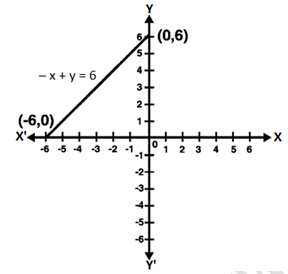(iv) It is given that
y = 2x
Put the value of x = 1
y = 2 × 1
y = 2
Put the value of x = 3
y = 2 × 3
y = 6(v) It is given that
3x + 5y = 15
5y = 15 – 3x
Put the value of x = 0
5y = 15 – 3 × 0
5y = 15 – 0
y = 15/5
y = 3
Put the value of x = 5
5y = 15 – 3 × 5
5y = 15 – 15
y = 0(vi) It is given that,
x/2 – y/3 = 2
(3x-2y)/6 = 2
3x – 2y = 12
y = ((3x - 12))/2
Put the value of x = 0
y = ((3×0 - 12))/2
y = (- 12)/2
y = - 6
Put the value of x = 4 ⇒
y = ((3×4 - 12))/2
y = ((12 - 12))/2
y = 0(vii) It is given that,
((x-2))/3 = y − 3
x – 2 = 3(y – 3)
x – 2 = 3y – 9
x = 3y – 7
Put the value of x = 5
x = 3y – 7
5 = 3y – 7
5 + 7 = 3y
12 = 3y
12/3 = y
y = 4
Put the value of x = 8
x = 3y – 7
8 = 3y – 7
8 + 7 = 3y
15 = 3y
y = 5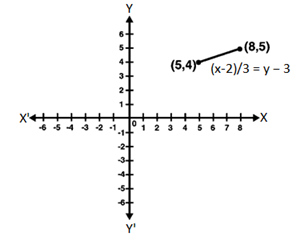(viii) It is given that,
2y = – x +1
2y = 1 – x
Put the value of x = 1
2y = 1 – x
2y = 1 – 1
2y = 0
y = 0
Put the value of x = 5
2y = 1 – x
2y = 1 – 5
2y = - 4
y = -2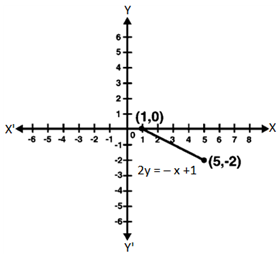Question 2: Give the equations of two lines passing through (3, 12). How many more such lines are there, and why?
Solution:
It is given that the value of x = 3 and value of y = 12.
4x – y = 0
3x – y + 3 = 0
Set of lines which are passing through (3, 12).
Hence, there are infinite lines passing through (3, 12).

Question 3: A three-wheeler scooter charges Rs. 15 for first kilometer and Rs. 8 each for every subsequent kilometre. For a distance of x km, an amount of Rs. y is paid. Write the linear equation representing the above information.
Solution:
It is given that, the total fare is Rs. y for covering the distance of ‘x’ km.
According to question,
y = 15 + 8(x – 1)
y = 15 + 8x – 8
y = 8x + 7
Hence the required equation according to question is y = 8x + 7.

Question 4: A lending library has a fixed charge for the first three days and an additional charge for each day thereafter. Aarushi paid Rs. 27 for a book kept for seven days. If fixed charges are Rs. x and per day charges are Rs y. Write the linear equation representing the above information.
Solution:
Let the fixed charges of library for 3 days will be Rs. x
Additional charges will be Rs. y
Total amount paid by Aarushi Rs. 27 for 7 days
x + (7 – 3) y = 27
x + 4y = 27
Hence the linear equation of the above equation is x + 4y = 27.

Question 5: A number is 27 more than the number obtained by reversing its digits. lf its unit’s and ten’s digit are x and y respectively, write the linear equation representing the statement.
Solution:
Let the once digit will be x and tans digit will be y.
Let us assume that the given number is in the form of 10y + x.
By reversing the digits of the number we get 10x + y.
According to question,
10y + x = 10x + y + 27
10y – y + x – 10x = 27
9y – 9x = 27
9(y – x) = 27
y – x = 3
x – y + 3 = 0
Hence, the required equation is x – y + 3 = 0.

Question 6: The Sum of a two digit number and the number obtained by reversing the order of its digits is 121. If units and ten’s digit of the number are x and y respectively, then write the linear equation representing the above statement.
Solution:
It is given that, the number is 10y + x.
By reversing of digits of the number, 10x + y
Sum of the two numbers is 121.
(10y + x) + (10x + y) = 121
10y + y + x + 10x = 121
11x + 11y = 121
x + y = 11
Hence, the required linear equation is x + y = 11.

Question 7: Plot the Points (3, 5) and (-1, 3) on a graph paper and verify that the straight line passing through the points, also passes through the point (1, 4).
Solution:
Let point A(1, 4), B(3, 5) and C(-1, 3)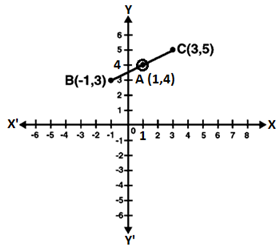Question 8:  From the choices given below, choose the equations whose graph is given in figure.
(i) y = x
(ii) x + y = 0
(iii) y = 2x
(iv) 2 + 3y = 7x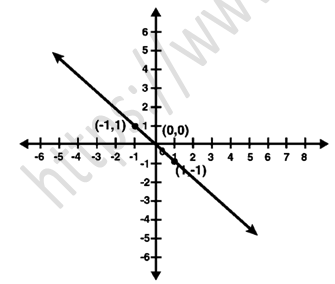Solution:
(i) For Co-ordinate (1, -1)
y = x
Put the value of x = 1 and y = -1 in equation
y = x
1 ≠ -1
L.H.S ≠ R.H.S
For Co-ordinate (-1, 1)
Put the value x = -1 and y = 1 in equation
y = x
-1 ≠ 1
L.H.S ≠ R.H.S
Hence, y = x does not represent the graph.

(ii) For Co-ordinate (1, -1)
x + y = 0
Put the value of x = 1 and y = -1 in equation
1 + (-1) = 0
0 = 0
L.H.S = R.H.S
For Co-ordinate (-1, 1)
Put the value of x = -1 and y = 1 in equation
(-1) + 1 = 0
0 = 0
L.H.S = R.H.S
Hence, x + y = 0 satisfy the equation for given solutions.

(iii) For Co-ordinate (1, -1)
y = 2x
Put the value of x = 1 and y = -1 in equation
-1 = 2(1)
-1 = 2
L.H.S ≠ R.H.S
For Co-ordinate (-1, 1)
Put the value of x = -1 and y = 1 in equation
1 = 2(-1)
1 = -2
L.H.S ≠ R.H.S
Hence, y = 2x does not represent the graph.

(iv) For Co-ordinate (1, -1)
2 + 3y = 7x
Put the value of x = 1 and y = -1 in equation
2 + 3(-1) = 7(1)
2 + (-3) = 7
2 - 3 = 7
-1 = 7
For Co-ordinate (-1, 1)
Put the value of x = -1 and y = 1 in equation
2 + 3(1) = 7(-1)
2 + 3 = -7
5 = -7
Hence, 2 + 3y = 7x does not represent the graph.

Question 9:  From the choices given below, choose the equation whose graph is given fig:
(i) y = x + 2
(ii) y = x – 2
(iii) y = – x + 2
(iv) x + 2y = 6Solution:
(i) For Coordinate (-1, 3)
y = x + 2
Put the value of x = -1 and y = 3 in equation
y = x + 2
3 = – 1 + 2
3 ≠ 1
L.H.S ≠ R.H.S
For Coordinate (2, 0)
Put the value of x = 2 and y = 0 in equation
y = x + 2
0 = 2 + 2
0 ≠ 4
L.H.S. ≠ R.H.S.
Hence, the equation y = x + 2 does not represent the graph.

(ii) For Coordinate (-1, 3)
y = x – 2
Put the value of, x = -1 and y = 3 in equation
3 = (-1) – 2
3 ≠ – 1 – 2
L.H.S ≠ R.H.S
For Coordinate (2, 0)
Put the value of, x = 2 and y = 0 in equation
0 = 2 – 2
0 = 0
L.H.S = R.H.S
Hence, the equation y = x - 2 does not represent the graph.

(iii) For Coordinate (-1, 3)
y = – x + 2
Put the value of, x = – 1 and y = 3 in equation
y = – x + 2
3 = – ( – 1 ) + 2
3 = 1 + 2
3 = 3
L.H.S = R.H.S
For Coordinate (2, 0)
Put the value of, x = 2 and y = 0 in equation
y = – x + 2
0 = – 2 + 2
0 = 0
L.H.S = R.H.S
Hence, the equation y = – x + 2 does represent the graph.

(iv) For Coordinate (-1, 3)
x + 2y = 6
Put the value of, x = – 1 and y = 3 in equation
x + 2y = 6
-1 + 2(3) = 6
-1 + 6 = 6
5 = 6
L.H.S ≠ R.H.S
For Coordinate (2, 0)
Put the value of, x = 2 and y = 0 in equation
x + 2y = 6
2 + 2(0) = 6
2 = 6
L.H.S ≠ R.H.S
Hence, the equation x + 2y = 6 does not represent the graph.

Question 10: If the point (2, -2) lies on the graph of linear equation, 5x + ky = 4, find the value of k.
Solution:
It is given that the point (2,-2) lies on the 5x + ky = 4 linear equation.
Put the value of x = 2 and y = -2 in equation.
5x + ky = 4
5 × 2 + (-2)k = 4
10 – 2k = 4
– 2k = 4 – 10
– 2k = – 6
k = (- 6)/(- 2)
k = 3
Hence, the value of k is 3.

Exercise 13.4

Question 1: Give the geometric representations of the following equations
(a) on the number line
(b) on the Cartesian plane:

(i) x = 2
(ii) y + 3 = 0
(iii) y = 3
(iv) 2x + 9 = 0
(v) 3x – 5 = 0
Solution:
(i) x = 2
Below is the representation of equation on the number line: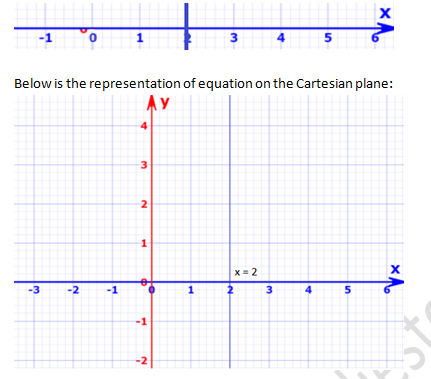(ii) y + 3 = 0
y = -3
Below is the representation of equation on the number line: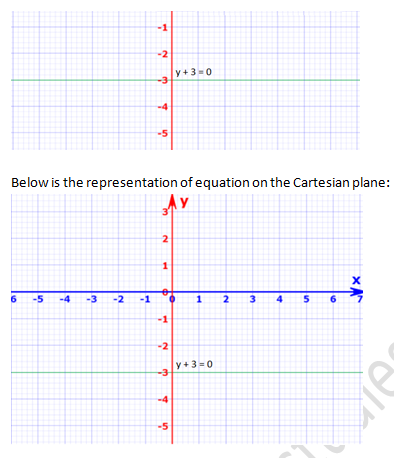(iii) y = 3
Below is the representation of equation on the number line: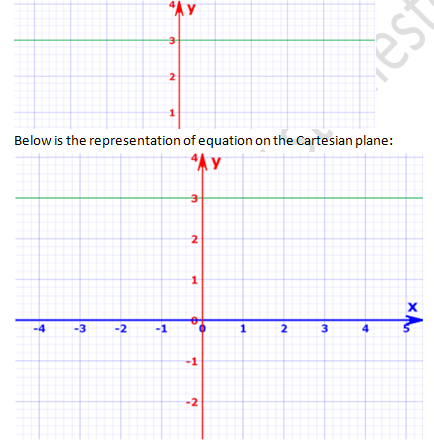(iv) 2x + 9 = 0
x = -9/2
Below is the representation of equation on the number line:(v) 3x – 5 = 0
x = 5/3
Below is the representation of equation on the number line: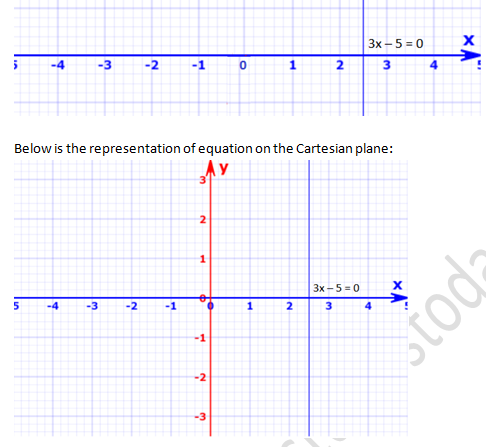Question 2 : Give the geometrical representation of 2x + 13 = 0 as an equation in
(i) one variable
(ii) two variables
Solution:
(i) 2x + 13 = 0
2x + 13 = 0
2x = -13
x = (-13)/2
x = -6.5
One variable graph:-(ii) 2x + 13 = 0
We can write it as:-
2x + 0y + 13 = 0
2x + 13 = 0
2x = -13
x = (-13)/2
x = -6.5
((-13)/2,0)
Two variable graph:-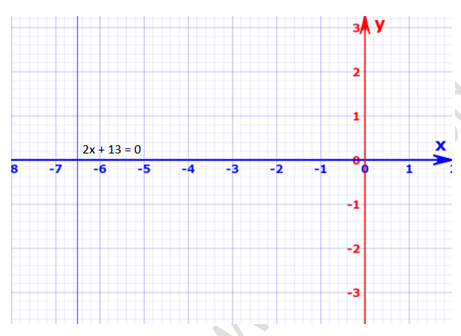Exercise VSAQs ........................

Question 1: Write the equation representing x-axis.
Solution:
The equation representing x-axis is y = 0.

Question 2: Write the equation representing y-axis.
Solution:
The equation representing y-axis is x = 0.

Question 3: Write the equation of a line passing through the point (0, 4) and parallel to x-axis.
Solution:
Coordination of x-axis is 0 and y-axis is 4.
Below is the representation of above coordinates.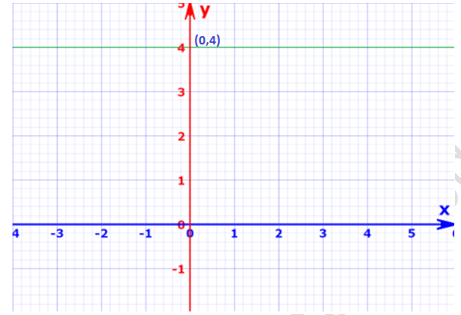Question 4: Write the equation of a line passing through the point (3, 5) and parallel to x-axis.
Solution:
Coordination of x-axis is 3 and y-axis is 5.
Below is the representation of above coordinates.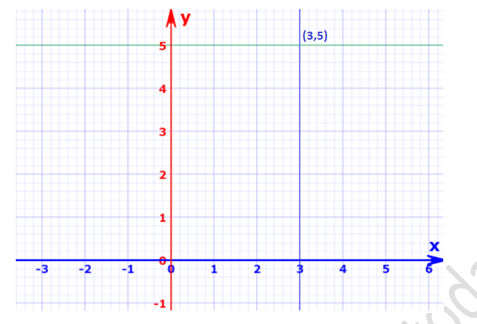Question 5: Write the equation of a line parallel to y-axis and passing through the point (-3, -7)
Solution:
Coordination of x-axis is -3 and y-axis is -7.
Below is the representation of above coordinates.